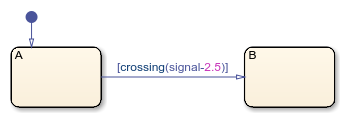# crossing

Detect rising or falling edge in data since last time step

Since R2021b

## Syntax

``tf = crossing(expression)``

## Description

example

````tf = crossing(expression)` returns 1 (`true`) if: The previous value of `expression` was positive and its current value is zero or negative.The previous value of `expression` was zero and its current value is nonzero.The previous value of `expression` was negative and its current value is zero or positive. Otherwise, the operator returns 0 (`false`). If `expression` changes value from positive to zero to negative or from negative to zero to positive at three consecutive time steps, the operator detects a single edge when the value of `expression` becomes zero.The argument `expression`: Must be a scalar-valued expressionCan combine chart input data, constants, nontunable parameters, continuous-time local data, and state data from Simulink® based statesCan include addition, subtraction, and multiplication of scalar variables, elements of a matrix, fields in a structure, or any valid combination of structure fields and matrix elements Index elements of a matrix by using numbers or expressions that evaluate to a constant integer.```

Note

Edge detection is supported only in Stateflow® charts in Simulink models.

## Examples

expand all

Transition out of state if the value of the input data `signal` crosses a threshold of 2.5.

`[crossing(signal-2.5)]`The edge is detected when the value of the expression `signal-2.5` becomes zero or changes sign.

## Tips

• The operator `crossing` imitates the behavior of a Trigger (Simulink) block with Trigger Type set to `either`.

• Edge detection for continuous-time local data and state data from Simulink based states is supported only in transition conditions.

• In atomic subcharts, map all input data that you use in edge detection expressions to input data or nontunable parameters in the main chart. Mapping these input data to output data, local data, or tunable parameters can result in undefined behavior.

## Version History

Introduced in R2021b